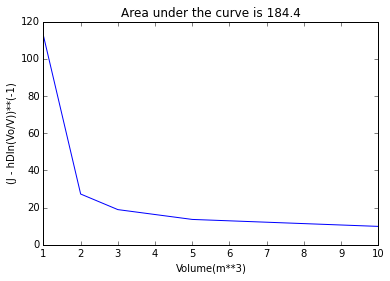# Chapter 8 - Membrane Separation Processes¶

## Page 460 Example 8.2¶

In :
%matplotlib inline
from matplotlib.pyplot import plot,title,xlabel,ylabel,show
from math import log

#From the gel polarisation model:
#J = (1/A)(dV/dt) = hD ln(Cg/Cf)
#Cf = Co(Vo/V)
#where Co and Vo are the initial concentration and volume,respectively and Cf and V are the values at subsequent times
#Combining these eq gives
#dV/dt = A(hDln(Cg/Co)-hDln(Vo/V))
V = [10, 5 ,3 ,2, 1]#
y = [9.90, 13.64, 18.92, 27.30, 112.40]
plot(V,y)
title("Area under the curve is 184.4")
xlabel("Volume(m**3)")
ylabel("(J - hDln(Vo/V))**(-1)")
show()

#(b)
Jo = 0.04*log(250/20)#
print"\n Jo = %.3f m/h"%(Jo)#
Jf = 0.04*log(250/200)#
print"\n Jf = %.3f   m/h"%(Jf)#
Jav = Jf + 0.27*(0.101-0.008)#
print"\n Jav = %.3f   m/h"%(Jav)#
#For the removal of 9m**3 filtrate in 4 hours
Area = (9/4)/Jav#
print"\n Area = %.3f  m**2"%(Area)#Jo = 0.099 m/h

Jf = 0.000   m/h

Jav = 0.025   m/h

Area = 79.650  m**2


## Page 462 Example 8.3¶

In :
from __future__ import division
from math import log
#It is assumed that Q0 is the volumetric flowrate of feed
# Q2 the volumetric flowrate of concentrate
#C0 the solute concentration in the feed
# C2 the solute concentration in the concentrate
# F the volumetric flowrate of membrane permeate
# A the required membrane area.
# It is also assumed that there is no loss of solute through the membrane.
Cl = 3#
while 1:
Clnew = Cl -(0.04-0.02*log(30/Cl))/(Cl**(-1)/50)#
if Clnew == Cl:
break#

Cl = Clnew#

print"\n Cl = %d kg/m**3"%(Cl)#
print"\n below this concentration the membrane flux is 0.04 m/h"

#This does not pose a constraint for the single stage as the concentration of solute C2 will be that of the final concentrate, 20 kg/m3.
#Conservation of solute gives:QoCo = Q2C2
#A fluid balance gives : Qo = F + Q2
#Combining these eq and substituting Known values:
A = (2.438/0.02)/log(30/20)#
print"\n A = %d m**2"%(A)#
#The tubular membranes to be used are available as 30 m**2modules.
print"\n the no of required modules are %d "%(A/30)#

#Part(b)

#Conservation of solute gives = QoCo = Q1C1 = Q2C2
#A fluid balance on stage 2 gives Q1 = Q2 + F2
#A fluid balance on stage 2 gives Q1 = Q2 +F2
#Substituting given values in above eqns
#2.5 = 1.25/C1 + 0.02A1ln(30/C1)
def a(C1):
A1 = (2.5-1.25/C1)/(0.02*log(30/C1))#
return A1
def b(C1):
A2 = (1.25/C1 - 0.0625)/0.00811
return A2

print"\n The procedure is to use trial and error to estimate the value of C1 that gives the optimum values of A1 and A2"
print"\n If C1 = 5kg/m**3 then A1 = %d m**2 and A2 = %d m**2"%(a(5),b(5))#
print"\n an arrangement of 3 modules −1 module is required."
print"\n\n\n  If C1 = 4 kg/m**3 then A1 = %dm**2 and A2 = %dm**2"%(a(4),b(4))#
print"\n an arrangement of 2 modules −1 module is almost sufficient."
print"\n\n\n   If C1 = 4.5 kg/m**3 then A1 = %dm**2 and A2 = %d m**2"%(a(4.5),b(4.5))#
print"\n an arrangement of 2 modules −1 module which meets the requirement"
print"\n\n This arrangement requires the minimum number of modules."

 Cl = 4 kg/m**3

below this concentration the membrane flux is 0.04 m/h

A = 300 m**2

the no of required modules are 10

The procedure is to use trial and error to estimate the value of C1 that gives the optimum values of A1 and A2

If C1 = 5kg/m**3 then A1 = 62 m**2 and A2 = 23 m**2

an arrangement of 3 modules −1 module is required.

If C1 = 4 kg/m**3 then A1 = 54m**2 and A2 = 30m**2

an arrangement of 2 modules −1 module is almost sufficient.

If C1 = 4.5 kg/m**3 then A1 = 58m**2 and A2 = 26 m**2

an arrangement of 2 modules −1 module which meets the requirement

This arrangement requires the minimum number of modules.